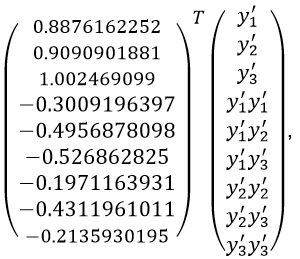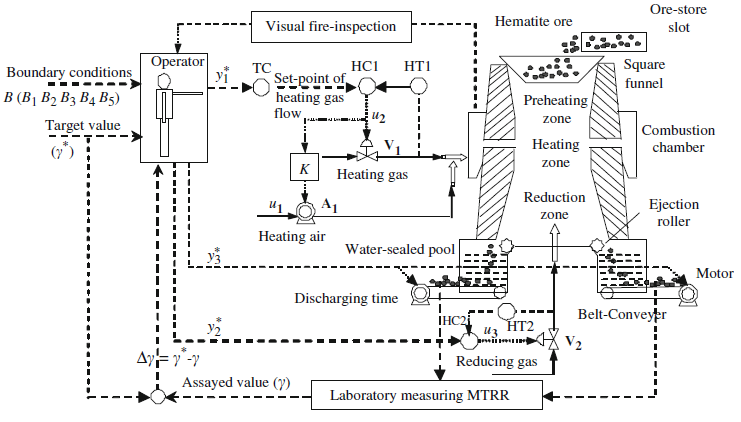# IEEE WCCI/CEC2020 Robust Optimization Competition

Time：2019-12-20Browse：280

#### Organized by

• Jinliang Ding (jlding@mail.neu.edu.cn, Northeastern University, Shenyang, China)

• Zhenan He (zhenan@scu.edu.cn, Sichuan University, Chengdu, China)

## Scope and Topics

In shaft furnace roasting process, the dynamics between Magnetic Tube Recovery Ratio (γ) and the temperature of combustion chamber (y1), the flow rate of reducing gas (y2), and the ore discharging time (y3) can be represented as:

γ = ƒ (y1, y2, y3, B, P),

(1)

where B is the boundary condition of production process and P is the process value of the shaft furnace. The relation between γ and y1, y2, y3 involves complex nonlinearities and strong couplings among process variables. From the view of metallurgy, it is difficult to construct such a dynamic model ƒ (•) through physical and chemical analysis. Instead, ƒ (•) is always approximated using neural networks based on historical production data or built as a case-based reasoning model according to domain experts’ empirical knowledge. This estimated model varies largely during the production process, where the uncertainty comes from three different types of sources. First, B and P vary largely and frequently during the production process, making the operation point change over time. Second, each of y1, y2 and y3 is maintained by a control loop through specific control action µ. However, the inaccurate setting of µ brings deviation between actual output and desired output. Third, there are inevitable model errors come from approximated neural network model and case-based reasoning model. Therefore, based on above discussion,  the precise model of ƒ (•) is difficult to obtain.

Since ƒ (•) exhibits strong uncertainty characteristics and its precise form cannot be deduced from metallurgy theory, the optimization of shaft furnace roasting process can be formulated as a single-objective robust optimization problem with the disturbance degree perturbed on the decision space (δ) and the noise added on the objective space (ε).

max γ = ƒ (y1+ δ1, y2 + δ2, y3+ δ3 ) + ε,

(2)

Due to the equipment constraints and production requirements, once updated, y1, y2 and y3 are kept consistent in the time period no matter how ƒ(•) changes over time. Three types of uncertainty source cause inaccurate function evaluation of (2), which can be represented as characteristics of δ and ε. Specially, δ is mainly determined by the first and the second types of uncertainty while ε is by the first and the third types of uncertainty. Based on above analysis, using historical production data, we characterize the range of each parameter as below: y1∈ [800, 1200], y2∈ [1500, 2700], y3∈ [300, 500], δ1 ∈ [-80, 80], δ2 ∈ [-240, 240], δ3 ∈ [-2, 2], and ε ∈ [-0.08, 0.02].

ƒ (•) is estimated by Support Vector Machine (SVM) and represented as:

γ =where y1’= (y1 - 800) / (1200 - 800), y2’= (y2 - 1500 ) / (2700 - 1500), and y3’= (y3 - 300 ) / (500 - 300 ) are normalized y1, y2, and y3, respectively. In this problem, the goal is to search for the robust optimal value of y1, y2, and y3, to maximize the value of γ in a certain time under the disturbance ε and δ.

As shown in Fig. 1, the shaft furnace carries out the high temperature-reduction roasting in order to turn the weakly magnetic ore into strongly one and obtain concentrated iron ore. Each set point is maintained by a control loop and cannot be changed more than once within two hours due to restriction of equipment condition. The imprecise roasting model causes the inaccurate function evaluation bringing inevitable deviation (ε) in objective values. Furthermore, the production boundary variations generate disturbance (δ) perturbed on each decision variable.Fig. 1. The shaft furnace roasting system

## Submission instructions

The participants are invited to submit their code to zhenan@scu.edu.cn. In performance evaluation, each submitted code will generate an approximate front consisting of 100 robust optimal solutions, which are tested under the disturbance. The number of generations for running each submitted code is 3,000. The number of testing times is set as 5,000. In each testing time, ε and δ are randomly sampled within their ranges. The objective value is recorded through the function evaluation under this specific disturbance setting. Among 5,000 times, the average and worst objective values are calculated and taken as the total performance of each algorithm (code).

### Reference

1.  Tianyou Chai, Jinliang Ding, and Fenghua Wu, “Hybrid intelligent control for optimal operation of shaft furnace roasting process,” Control Engineering Practice, vol. 19, no. 3, pp. 264-275, 2011.

2. Zhenan He, Gary G. Yen, and Jiancheng Lv,“Evolutionary Multi-objective Optimization with Robustness Enhancement,” IEEE Transactions on Evolutionary Computation, early access, DOI:  10.1109/TEVC.2019.2933444, 2019.

3. Zhenan He, Gary G. Yen, and Zhang Yi, “Robust Multiobjective Optimization via Evolutionary Algorithms,” IEEE Transactions on Evolutionary Computation, vol. 23, no. 2, pp. 316-330, 2019.